Want to share your content on R-bloggers? click here if you have a blog, or here if you don't.

## Background

At the AACC meeting recently, there was an enthusiastic discussion of standardization of reporting for serum protein electrophoresis (SPEP) presented by a working group headed up by Dr. Chris McCudden and Dr. Ron Booth, both of the University of Ottawa. One of the discussions pertained to how monoclonal bands, especially small ones, should be integrated. While many use the default manual vertical gating or “drop” method offered by Sebia's Phoresis software, Dr. David Keren was discussing the value of tangent skimming as a more repeatable and effective means of monoclonal protein quantitation. He was also discussing some biochemical approaches distinguishing monoclonal proteins from the background gamma proteins.

The drop method is essentially an eye-ball approach to where the peak starts and ends and is represented by the vertical lines and the enclosed shaded area.The tangent skimming approach is easier to make reproducible. In the mass spectrometry world it is a well-developed approach with a long history and multiple algorithms in use. This is apparently the book. However, when tangent skimming is employed in SPEP, unless I am mistaken, it seems to be done by eye. The integration would look like this: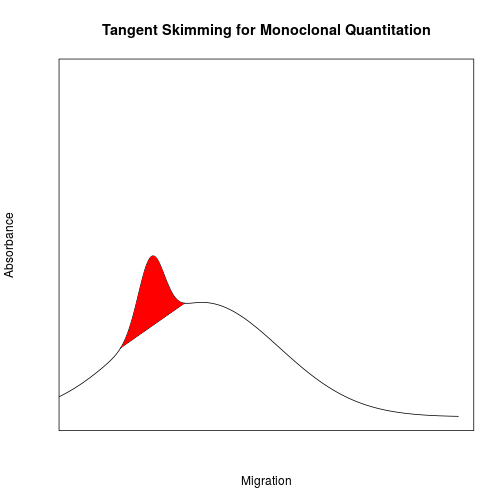During the discussion it was point out that peak deconvolution of the monoclonal protein from the background gamma might be preferable to either of the two described procedures. By this I mean integration as follows: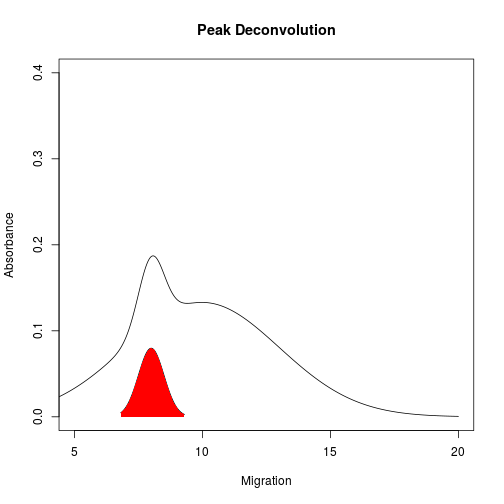There was discussion this procedure is challenging for number of reasons. Further, it should be noted that there will only likely be any clinical value in a deconvolution approach when the concentration of the monoclonal protein is low enough that manual integration will show poor repeatability, say < 5 g/L = 0.5 g/dL.

## Easy Peaks

Fitting samples with larger monoclonal peaks is fairly easy. Fitting tends to converge nicely and produce something meaningful. For example, using the approach I am about to show below, an electropherogram like this: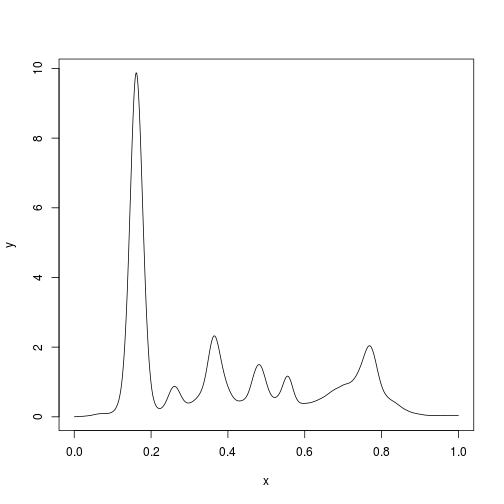with a gamma region looking like this: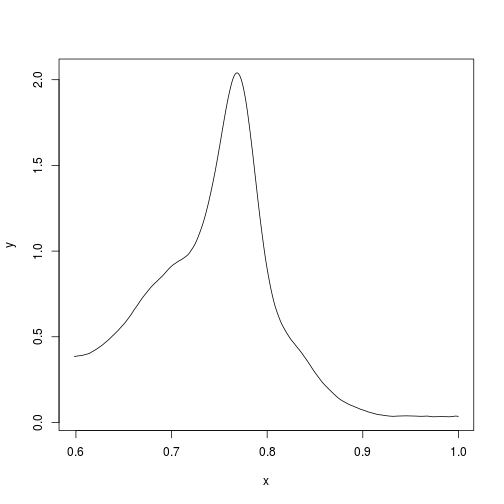can be deconvoluted with straightforward non-linear regression (and no baseline subtraction) to yield this: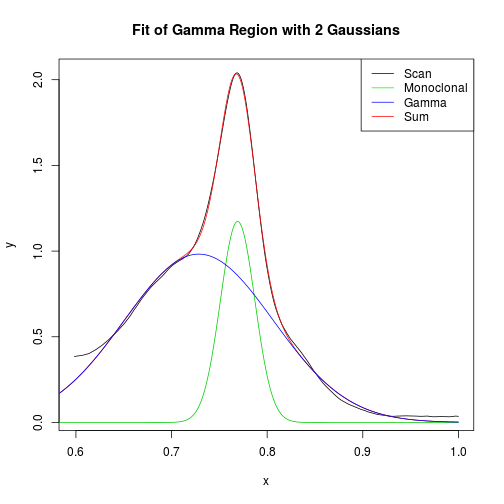and the area of the green monoclonal peak is found to be 5.3%.

## More Difficult Peaks

What is more challenging is the problem of small monoclonals buried in normal $$\gamma$$-globulins. These could be difficult to integrate using a tangent skimming approach, particularly without image magnification. For the remainder of this post we will use a gel with a small monoclonal in the fast gamma region shown at the arrow.### Getting the Data

EP data can be extracted from the PDF output from any electrophoresis software. This is not complicated and can be accomplished with pdf2svg or Inkscape and some Linux bash scripting. I'm sure we can get it straight from the instrument but it is not obvious to me how to do this. One could also rescan a gel and use ImageJ to produce a densitometry scan which is discussed in the ImageJ documentation and on YouTube. ImageJ also has a macro language for situations where the same kind of processing is done repeatedly.

### Smoothing

The data has 10284 pairs of (x,y) data. But if you blow up on it and look carefully you find that it is a series of staircases.

plot(y~x, data = head(ep.data,100), type = "o", cex = 0.5)It turns out that this jaggedness significantly impairs attempts to numerically identify the peaks and valleys. So, I smoothed it a little using the handy rle() function to identify the midpoint of each step. This keeps the total area as close to its original value as possible–though this probably does not matter too much.

ep.rle <- rle(ep.data$y) stair.midpoints <- cumsum(ep.rle$lengths) - floor(ep.rle$lengths/2) ep.data.sm <- ep.data[stair.midpoints,] plot(y~x, data = head(ep.data,300), type = "o", cex = 0.5) points(y~x, data = head(ep.data.sm,300), type = "o", cex = 0.5, col = "red")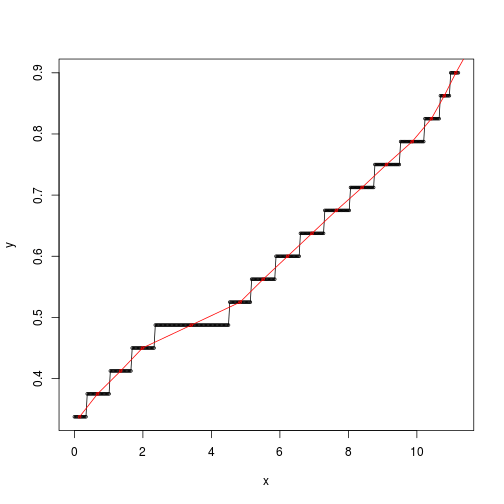Now that we are satisfied that the new data is OK, I will overwrite the original dataframe. ep.data <- ep.data.sm ### Transformation The units on the x and y-axes are arbitrary and come from page coordinates of the PDF. We can normalize the scan by making the x-axis go from 0 to 1 and by making the total area 1. library(Bolstad) #A package containing a function for Simpon's Rule integration ep.data$x <- ep.data$x/max(ep.data$x)
A.tot <- sintegral(ep.data$x,ep.data$y)$value ep.data$y <- ep.data$y/A.tot #sanity check sintegral(ep.data$x,ep.data$y)$value

##  1

plot(y~x, data = ep.data, type = "l")### Find Extrema

Using the findPeaks function from the quantmod package we can find the minima and maxima:

library(quantmod)
ep.max <- findPeaks(ep.data$y) plot(y~x, data = ep.data, type = "l", main = "Maxima") abline(v = ep.data$x[ep.max], col = "red", lty = 2)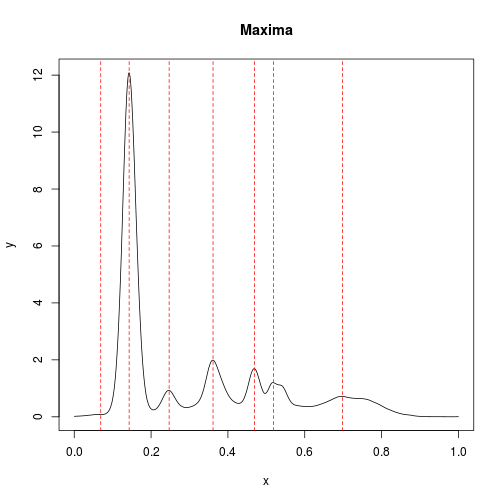ep.min <- findValleys(ep.data$y) plot(y~x, data = ep.data, type = "l", main = "Minima") abline(v = ep.data$x[ep.min], col = "blue", lty = 2)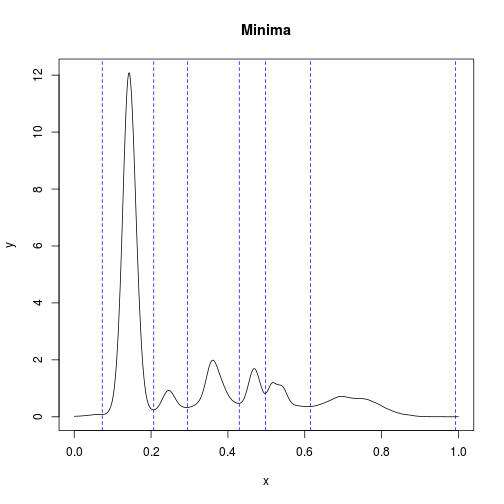Not surprisingly, there are some extraneous local extrema that we do not want. I simply manually removed them. Generally, this kind of thing could be tackled with more smoothing of the data prior to analysis.

ep.max <- ep.max[-1]
ep.min <- ep.min[-c(1,length(ep.min))]

## Fitting

Now it's possible with the nls() function to fit the entire SPEP with a series of Gaussian curves simultaneously. It works just fine (provided you have decent initial estimates of $$\mu_i$$ and $$\sigma_i$$) but there is no particular clinical value to fitting the albumin, $$\alpha_1$$, $$\alpha_2$$, $$\beta_1$$ and $$\beta_2$$ domains with Gaussians. What is of interest is separately quantifying the two peaks in $$\gamma$$ with two separate Gaussians so let's isolate the $$\gamma$$ region based on the location of the minimum between $$\beta_2$$ and $$\gamma$$.

### Isolate the $$\gamma$$ Region

gamma.ind <- max(ep.min):nrow(ep.data)
gamma.data <- data.frame(x = ep.data$x[gamma.ind], y = ep.data$y[gamma.ind])
plot(y ~ x, gamma.data, type  = "l")### Attempt Something that Ultimately Does Not Work

At first I thought I could just throw two normal distributions at this and it would work. However, it does not work well at all and this kind of not-so-helpful fit turns out to happen a fair bit. I use the nls() function here which is easy to call. It requires a functional form which I set to be:

$y = C_1 \exp\Big(-{\frac{(x-\mu_1)^2}{2\sigma_1^2}}\Big) + C_2 \exp \Big({-\frac{(x-\mu_2)^2}{2\sigma_2^2}}\Big)$

where $$\mu_1$$ is the $$x$$ location of the first peak in $$\gamma$$ and $$\mu_2$$ is the $$x$$ location of the second peak in $$\gamma$$. The estimates of $$\sigma_1$$ and $$\sigma_2$$ can be obtained by trying to estimate the full-width-half-maximum (FWHM) of the peaks, which is related to $$\sigma$$ by

$FWHM_i = 2 \sqrt{2\ln2} \times \sigma_i = 2.355 \times \sigma_i$

I had to first make a little function that returns the respective half-widths at half-maximum and then uses them to estimate the $$FWHM$$. Because the peaks are poorly resolved, it also tries to get the smallest possible estimate returning this as FWHM2.

FWHM.finder <- function(ep.data, mu.index){
peak.height <- ep.data$y[mu.index] fxn.for.roots <- ep.data$y - peak.height/2
indices <- 1:nrow(ep.data)
root.indices <- which(diff(sign(fxn.for.roots))!=0)
tmp <- c(root.indices,mu.index) %>% sort
tmp2 <- which(tmp == mu.index)
first.root <- root.indices[tmp2 -1]
second.root <- root.indices[tmp2]
HWHM1 <- ep.data$x[mu.index] - ep.data$x[first.root]
HWHM2 <- ep.data$x[second.root] - ep.data$x[mu.index]
FWHM <- HWHM2 + HWHM1
FWHM2 = 2*min(c(HWHM1,HWHM2))
return(list(HWHM1 = HWHM1,HWHM2 = HWHM2,FWHM = FWHM,FWHM2 = FWHM2))
}

The peak in the $$\gamma$$ region was obtained previously:

plot(y ~ x, gamma.data, type  = "l")
gamma.max <- findPeaks(gamma.data$y) abline(v = gamma.data$x[gamma.max])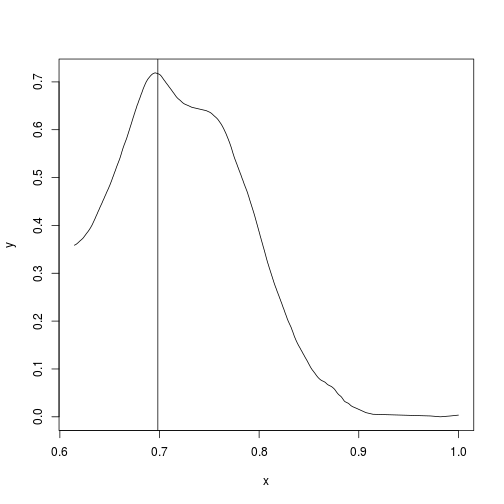and from them $$\mu_1$$ is determined to be 0.7. We have to guess where the second peak is, which is at about $$x=0.75$$ and has an index of 252 in the gamma.data dataframe.

gamma.data[252,]

##             x         y
## 252 0.7487757 0.6381026

#append the second peak
gamma.max <- c(gamma.max,252)
gamma.mu <- gamma.data$x[gamma.max] gamma.mu ##  0.6983350 0.7487757 plot(y ~ x, gamma.data, type = "l") abline(v = gamma.data$x[gamma.max])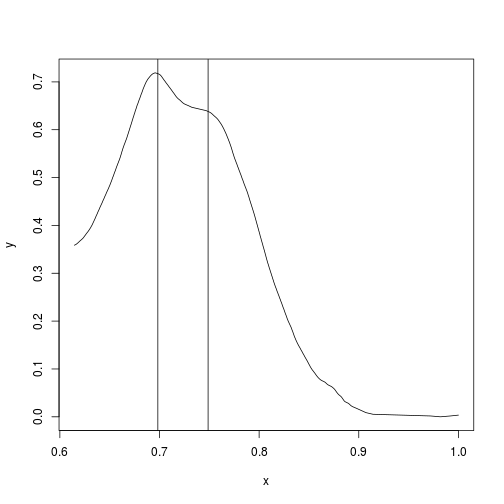Now we can find the estimates of the standard deviations:

#find the FWHM estimates of sigma_1 and sigma_2:
FWHM <- lapply(gamma.max, FWHM.finder, ep.data = gamma.data)
gamma.sigma <- unlist(sapply(FWHM, '[', 'FWHM2'))/2.355

The estimates of $$\sigma_1$$ and $$\sigma_2$$ are now obtained. The estimates of $$C_1$$ and $$C_2$$ are just the peak heights.

peak.heights <- gamma.data$y[gamma.max] We can now use nls() to determine the fit. fit <- nls(y ~ (C1*exp(-(x-mean1)**2/(2 * sigma1**2)) + C2*exp(-(x-mean2)**2/(2 * sigma2**2))), data = gamma.data, start = list(mean1 = gamma.mu, mean2 = gamma.mu, sigma1 = gamma.sigma, sigma2 = gamma.sigma, C1 = peak.heights, C2 = peak.heights), algorithm = "port") Determining the fitted values of our unknown coefficients: dffit <- data.frame(x=seq(0, 1 , 0.001)) dffit$y <- predict(fit, newdata=dffit)

fit.sum <- summary(fit)
fit.sum #show the fitted coefficients

##
## Formula: y ~ (C1 * exp(-(x - mean1)^2/(2 * sigma1^2)) + C2 * exp(-(x -
##     mean2)^2/(2 * sigma2^2)))
##
## Parameters:
##         Estimate Std. Error t value Pr(>|t|)
## mean1  0.7094793  0.0003312 2142.23   <2e-16 ***
## mean2  0.7813900  0.0007213 1083.24   <2e-16 ***
## sigma1 0.0731113  0.0002382  306.94   <2e-16 ***
## sigma2 0.0250850  0.0011115   22.57   <2e-16 ***
## C1     0.6983921  0.0018462  378.29   <2e-16 ***
## C2     0.0819704  0.0032625   25.12   <2e-16 ***
## ---
## Signif. codes:  0 '***' 0.001 '**' 0.01 '*' 0.05 '.' 0.1 ' ' 1
##
## Residual standard error: 0.01291 on 611 degrees of freedom
##
## Algorithm "port", convergence message: both X-convergence and relative convergence (5)

coef.fit <- fit.sum$coefficients[,1] mu.fit <- coef.fit[1:2] sigma.fit <- coef.fit[3:4] C.fit <- coef.fit[5:6] And now we can plot the fitted results against the original results: #original plot(y ~ x, data = gamma.data, type = "l", main = "This is Garbage") #overall fit lines(y ~ x, data = dffit, col ="red", cex = 0.2) legend("topright", lty = c(1,1,1), col = c("black", "green", "blue","red"), c("Scan", "Monoclonal", "Gamma", "Sum")) #components of the fit for(i in 1:2){ x <- dffit$x
y <- C.fit[i] *exp(-(x-mu.fit[i])**2/(2 * sigma.fit[i]**2))
lines(x,y, col = i + 2)
}And this is garbage. The green curve is supposed to be the monoclonal peak, the blue curve is supposed to be the $$\gamma$$ background, and the red curve is their sum, the overall fit. This is a horrible failure.

Subsequently, I tried fixing the locations of $$\mu_1$$ and $$\mu_2$$ but this also yielded similar nonsensical fitting. So, with a lot of messing around trying different functions like the lognormal distribution, the Bi-Gaussian distribution and the Exponentially Modified Gaussian distribution, and applying various arbitrary weighting functions, and simultaneously fitting the other regions of the SPEP, I concluded that nothing could predictably produce results that represented the clinical reality.

I thought maybe the challenge to obtain a reasonable fit related to the sloping baseline, so I though I would try to remove it. I will model the baseline in the most simplistic manner possible: as a sloped line.

## Baseline Removal

I will arbitrarily define the tail of the $$\gamma$$ region to be those values having $$y \leq 0.02$$. Then I will connect the first (x,y) point from the $$\gamma$$ region and connect it to the tail.

gamma.tail <- filter(gamma.data, y <= 0.02)
baseline.data <- rbind(gamma.data[1,],gamma.tail)
names(baseline.data) <- c("x","y")
baseline.fun <- approxfun(baseline.data)
plot(y~x, data = gamma.data, type = "l")
lines(baseline.data$x,baseline.fun(baseline.data$x), col = "blue")Now we can define a new dataframe gamma.no.base that has the baseline removed:

gamma.no.base <- data.frame(x = gamma.data$x, y = gamma.data$y - baseline.fun(gamma.data$x)) plot(y~x, data = gamma.data, type = "l") lines(y ~ x, data = gamma.no.base, lty = 2) gamma.max <- findPeaks(gamma.no.base$y)[1:2] #rejects a number of extraneous peaks
abline(v = gamma.no.base$x[gamma.max])The black is the original $$\gamma$$ and the dashed has the baseline removed. This becomes and easy fit. #Estimate the Ci peak.heights <- gamma.no.base$y[gamma.max]
#Estimate the mu_i
gamma.mu <- gamma.no.base$x[gamma.max] #the same values as before #Estimate the sigma_i from the FWHM FWHM <- lapply(gamma.max, FWHM.finder, ep.data = gamma.no.base) gamma.sigma <- unlist(sapply(FWHM, '[', 'FWHM2'))/2.355 #Perform the fit fit <- nls(y ~ (C1*exp(-(x-mean1)**2/(2 * sigma1**2)) + C2*exp(-(x-mean2)**2/(2 * sigma2**2))), data = gamma.no.base, start = list(mean1 = gamma.mu, mean2 = gamma.mu, sigma1 = gamma.sigma, sigma2 = gamma.sigma, C1 = peak.heights, C2 = peak.heights), algorithm = "port") #Plot the fit dffit <- data.frame(x=seq(0, 1 , 0.001)) dffit$y <- predict(fit, newdata=dffit)
fit.sum <- summary(fit)
coef.fit <- fit.sum$coefficients[,1] mu.fit <- coef.fit[1:2] sigma.fit <- coef.fit[3:4] C.fit <- coef.fit[5:6] plot(y ~ x, data = gamma.no.base, type = "l") legend("topright", lty = c(1,1,1), col = c("black", "green", "blue","red"), c("Scan", "Monoclonal", "Gamma", "Sum")) lines(y ~ x, data = dffit, col ="red", cex = 0.2) for(i in 1:2){ x <- dffit$x
y <- C.fit[i] *exp(-(x-mu.fit[i])**2/(2 * sigma.fit[i]**2))
lines(x,y, col = i + 2)
}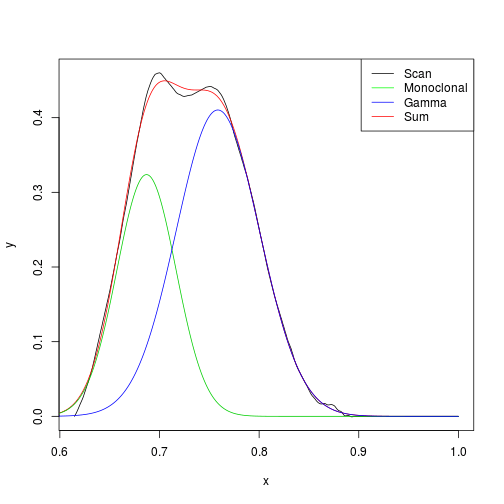Lo and behold…something that is not completely insane. The green is the monoclonal, the blue is the $$\gamma$$ background and the red is their sum, that is, the overall fit. A better fit could now we sought with weighting or with a more flexible distribution shape. In any case, the green peak is now easily determined. Since

$\int_{-\infty}^{\infty} C_1 \exp\Big(-{\frac{(x-\mu_1)^2}{2\sigma_1^2}}\Big)dx = \sqrt{2\pi}\sigma C_1$

A.mono <- sqrt(2*pi)*sigma.fit*C.fit %>% unname()
A.mono <- round(A.mono,3)
A.mono

## sigma1
##  0.024

So this peak is 2.4% of the total area. Now, of course, this assumes that nothing under the baseline is attributable to the monoclonal peak and all belongs to normal $$\gamma$$-globulins, which is very unlikely to be true. However, the drop and tangent skimming methods also make assumptions about how the area under the curve contributes to the monoclonal protein. The point is to try to do something that will produce consistent results that can be followed over time. Obviously, if you thought there were three peaks in the $$\gamma$$-region, you'd have to set up your model accordingly.

There are obviously better ways to model the baseline because this approach of a linear baseline is not going to work in situations where, for example, there is a small monoclonal in fast $$\gamma$$ dwarfed by normal $$\gamma$$-globulins. That is, like this: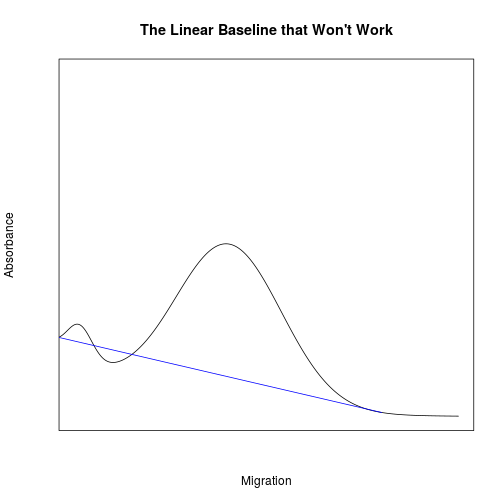Something curvilinear or piecewise continuous and flexible enough for more circumstances is generally required.

There is also no guarantee that baseline removal, whatever the approach, is going to be a good solution in other circumstances. Given the diversity of monoclonal peak locations, sizes and shapes, I suspect one would need a few different approaches for different circumstances.

## Conclusions

• The data in the PDFs generated by EP software are processed (probably with splining or similar) followed by the stair-stepping seen above. It would be better to work with raw data from the scanner.

• Integrating monoclonal peaks under the $$\gamma$$ baseline (or $$\beta$$) is unlikely to be a one-size-fits all approach and may require application of a number of strategies to get meaningful results.

• Basline removal might be helpful at times.
• Peak integration will require human adjudication.

• While most monoclonal peaks show little skewing, better fitting is likely to be obtained with distributions that afford some skewing.

• MASSFIX may soon make this entire discussion irrelevant.

Parting Thought

On the matter of fitting

In bringing many sons and daughters to glory, it was fitting that God, for whom and through whom everything exists, should make the pioneer of their salvation perfect through what he suffered.

Heb 2:10